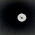# [Maths Tricks]Fast calculation in maths tricks tutorial 2

11:42 PM
1We already shared Part 1/[Tutorial 1 click here to go] Maths Tricks|Aptitude Tricks|Speed Maths|Fast Calculation|mental maths tricks,maths. So in this Tutorial 2 we gonna share maths tricks and shortcut key to do fast mental calculation here.

### How to find calculate remainder on dividing by 27 and 37 in 3 secs

Question 1:  find the remainder  45286789 on dividing by 27 and 37 repectively
Rule :1
step 1:split that  45286789 as 45.... 286....789
step 2:Some all digits above separated digits ---> 45+286+789= 1120
Again split  1120 as 1...120
Add above separated digits --> 1+120 = 121(Repeat the step 2 until get as 3 digit number)
Step 3: Now divide 121 by 27 we get remainder as 121/27 = 4 as Q(quotient) ; 13 as Remainder (108+13=121)
Now divide 121 by 37 we get remainder as 121/37 = 3 as Quotient ; 10 as Remainder
(111+10=121)

verification/Proof
45286789/27 =1677288 as Q  ; 13 as remainder  ( 45286776  + 13 =45286789)
45286789/37 =1223967 as Q  ; 10 as remainder   ( 45286779 + 10 =45286789)

### Tricks to find remainder easily by dividing 27 and 37

Question 2:  find the remainder  34568278 on dividing by 27 and 37 repectively
Rule :1
step 1:split that  45286789 as 34.... 568....278
step 2:Some all digits above separated digits ---> 34+568+278= 880
now no need to split  880 because we got the number as three digit
Add above separated digits --> 0+880 = 880 only
Step 3: Now divide 880 by 27 we get remainder as 880/27 = 32 as Q(quotient) ; 16 as Remainder (864+16=880)
Now divide 121 by 37 we get remainder as 121/37 = 23 as Q(quotient) ; 29 as Remainder
(851+29=880)

verification/Proof
34568278 /27 =1280306 as Q  ; 13 as remainder  ( 34568262 + 16=34568278 )
34568278 /37 = 934277  as Q  ; 29 as remainder  ( 34568249 + 29 =34568278 )

## Tutorial Part 5 Click here to go

1.